😎手撕吊打面试官系列面试题

## js基础

### 1. 用js打印一个乘法表

``````console.log(`
1*1=1
2*1=2 2*2=4
3*1=3 3*2=6 3*3=9
4*1=4 4*2=8 4*3=12 4*4=16
5*1=5 5*2=10 5*3=15 5*4=20 5*5=25
6*1=6 6*2=12 6*3=18 6*4=24 6*5=30 6*6=36
7*1=7 7*2=14 7*3=21 7*4=28 7*5=35 7*6=42 7*7=49
8*1=8 8*2=16 8*3=24 8*4=32 8*5=40 8*6=48 8*7=56 8*8=64
9*1=9 9*2=18 9*3=27 9*4=36 9*5=45 9*6=54 9*7=63 9*8=72 9*9=81
`)  ``````

### 斐波那契数列函数(30以内)

``````const arr = [0,1,1,2,3,5,8,13,21,34,55,89,144,233,377,610,987,1597,2584,4181,6765,10946,17711,28657,46368,75025,121393,196418,317811,514229,832040,1346269]
var fib = function(N) {
return arr[N]
}  ``````

### 不用sort实现排序，比如输入[3,2,6,9,1,4,8] 返回排序后的数组

``````function mySort(arr){
return [1,2,3,6,8,9]
}  ``````

### 请js实现一个permute函数，输入数字123， 打印出这三个数字的全排列

``````function permute(){
return [
[1,2,3],
[1,3,2],
[2,1,3],
[2,3,1],
[3,1,2],
[3,2,1],
]
}  ``````

### 代码笔试题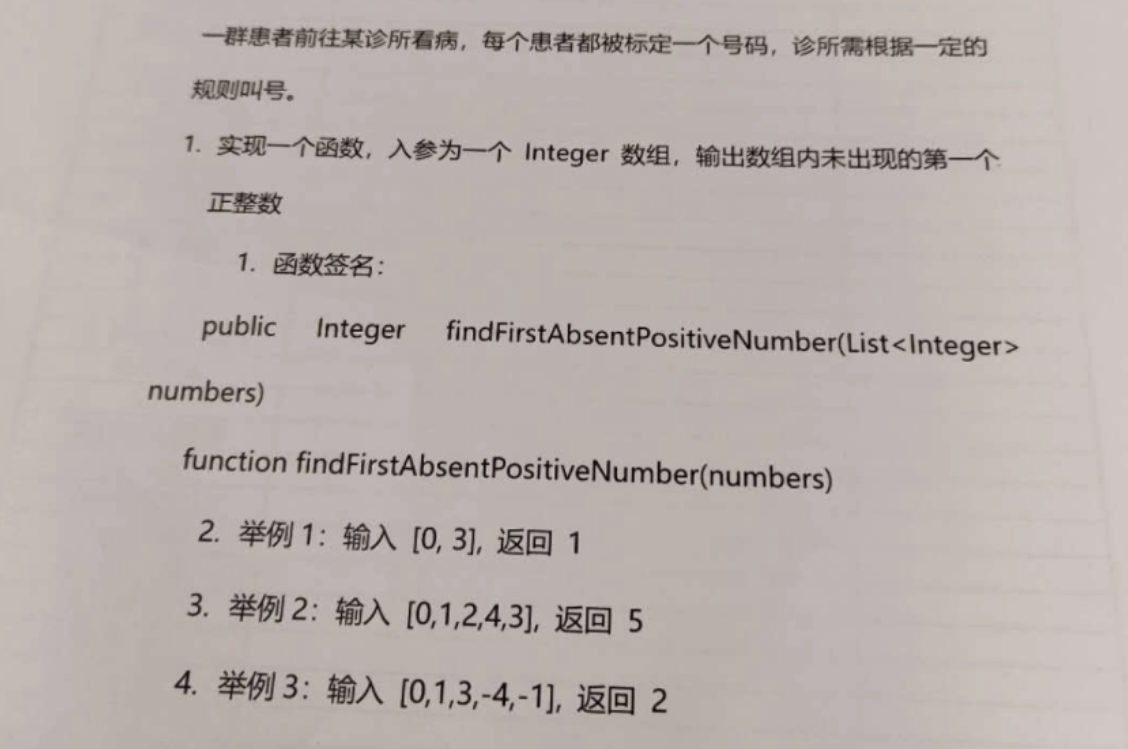根据输入的特点，判断输出，主要是如何高效的筛选过滤条件

``````function findFirstAbsentPositiveNumber(arr) {
if (arr === 0) {
if (arr === 1) {
if (arr === 2) {
return 5
}
return 2
}
return 1
}
return 1
}  ``````

## 框架和最佳实践

### 平时设计过组件吗

``"★★★★★☆☆☆☆☆".slice(5 - rate, 10 - rate);  ``

### 谈一下你对SPA的理解

SPA一词源于拉丁文“Solus Par Agula”（SPA是指利用水资源结合沐浴、按摩、涂抹保养品和香熏来促进新陈代谢，满足人体视觉、味觉、触觉、嗅觉和思考达到一种身心畅快的享受。日程程序员工作比较辛苦，尤其肩颈手腕会僵硬，经常SPA会缓解这些症状，前端工程师必备，需要经常去SPA.

## 部门需求太多，如何协调加班和家庭秃头王路飞
5个月前
webpack5手撸vue2脚手架
webpack5手撸vue相信工作个12年的小伙伴们在面试的时候多多少少怕被问到关于webpack方面的知识，本菜鸟最近闲来无事，就尝试了手撸了下vue2的脚手架，第一次发帖实在是没有经验，望海涵。languageJavaScript"name":"vuecliversion2","version":"1.0.0","desc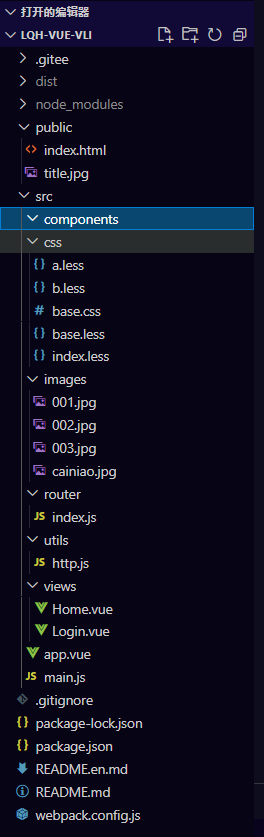blmius
1年前
MySQL:[Err] 1292 - Incorrect datetime value: ‘0000-00-00 00:00:00‘ for column ‘CREATE_TIME‘ at row 1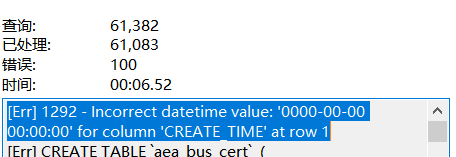光头强的博客
5个月前
Java面向对象试题
1、请创建一个Animal动物类，要求有方法eat（）方法，方法输出一条语句“吃东西”。创建一个接口A，接口里有一个抽象方法fly（）。创建一个Bird类继承Animal类并实现接口A里的方法输出一条有语句“鸟儿飞翔”，重写eat（）方法输出一条语句“鸟儿吃虫”。在Test类中向上转型创建b对象，调用eat方法。然后向下转型调用eat（）方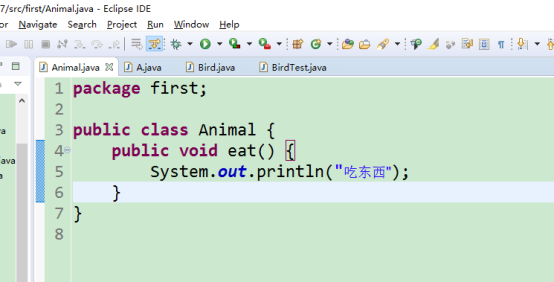刚刚好
5个月前
css问题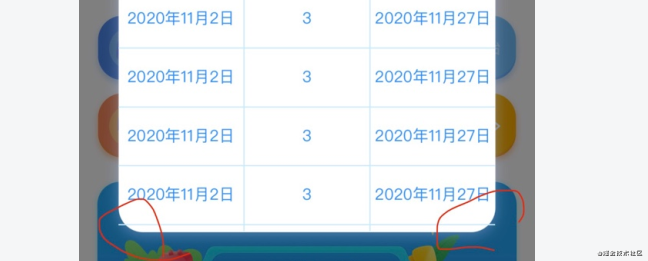小森森
5个月前晴空闲云
5个月前
css中box-sizing解放盒子实际宽高计算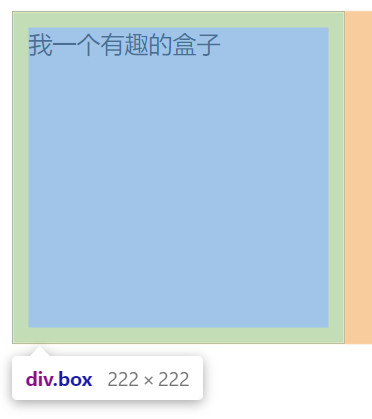艾木酱
5个月前

MemFireCloud是一款提供云数据库，用户可以创建云数据库，并对数据库进行管理，还可以对数据库进行备份操作。它还提供后端即服务，用户可以在1分钟内新建一个应用，使用自动生成的API和SDK，访问云数据库、对象存储、用户认证与授权等功能，可专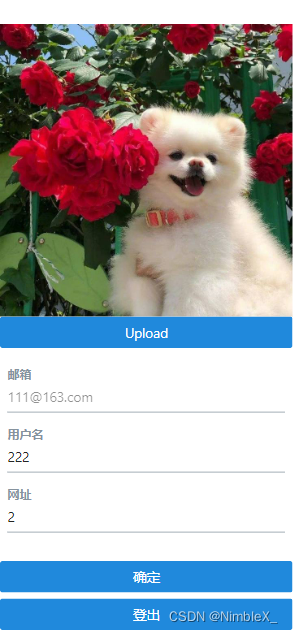天翼云开发者社区
1个月前天翼云开发者社区
1个月前
NVIDIA安培架构下MIG技术分析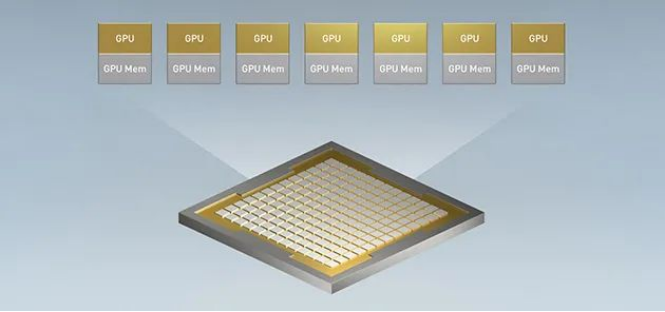helloworld_28799839
5个月前

Javascript判断对象是否为空jsObject.keys(myObject).length0经常使用的三元运算我们经常遇到处理表格列状态字段如status的时候可以用到vueSouleigh ✨
Lv1
FE
Coding...💻
123

30

48